Series Quiz IV, Non Verbal Reasoning

# Series Quiz IV, Non Verbal Reasoning - SSC CGL

Test Description

## 20 Questions MCQ Test General Intelligence and Reasoning for SSC CGL - Series Quiz IV, Non Verbal Reasoning

Series Quiz IV, Non Verbal Reasoning for SSC CGL 2023 is part of General Intelligence and Reasoning for SSC CGL preparation. The Series Quiz IV, Non Verbal Reasoning questions and answers have been prepared according to the SSC CGL exam syllabus.The Series Quiz IV, Non Verbal Reasoning MCQs are made for SSC CGL 2023 Exam. Find important definitions, questions, notes, meanings, examples, exercises, MCQs and online tests for Series Quiz IV, Non Verbal Reasoning below.
Solutions of Series Quiz IV, Non Verbal Reasoning questions in English are available as part of our General Intelligence and Reasoning for SSC CGL for SSC CGL & Series Quiz IV, Non Verbal Reasoning solutions in Hindi for General Intelligence and Reasoning for SSC CGL course. Download more important topics, notes, lectures and mock test series for SSC CGL Exam by signing up for free. Attempt Series Quiz IV, Non Verbal Reasoning | 20 questions in 40 minutes | Mock test for SSC CGL preparation | Free important questions MCQ to study General Intelligence and Reasoning for SSC CGL for SSC CGL Exam | Download free PDF with solutions
 1 Crore+ students have signed up on EduRev. Have you?
Series Quiz IV, Non Verbal Reasoning - Question 1

### Directions to Solve Each of the following questions consists of five figures marked A, B, C, D and E called the Problem Figures followed by five other figures marked 1, 2, 3, 4 and 5 called the Answer Figures. Select a figure from amongst the Answer Figures which will continue the same series as established by the five Problem Figures. Question - Select a figure from amongst the Answer Figures which will continue the same series as established by the five Problem Figures.Problem Figures:                          Answer Figures: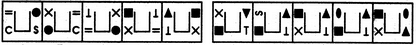(A)     (B)     (C)     (D)     (E)       (1)     (2)     (3)     (4)     (5)

Detailed Solution for Series Quiz IV, Non Verbal Reasoning - Question 1
In each step, the symbols move in the order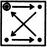and the symbol that reaches the upper-left position gets replaced by a new one.
Series Quiz IV, Non Verbal Reasoning - Question 2

### Directions to Solve Each of the following questions consists of five figures marked A, B, C, D and E called the Problem Figures followed by five other figures marked 1, 2, 3, 4 and 5 called the Answer Figures. Select a figure from amongst the Answer Figures which will continue the same series as established by the five Problem Figures. Question - Select a figure from amongst the Answer Figures which will continue the same series as established by the five Problem Figures.Problem Figures:                          Answer Figures: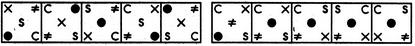(A)     (B)     (C)     (D)     (E)       (1)     (2)     (3)     (4)     (5)

Detailed Solution for Series Quiz IV, Non Verbal Reasoning - Question 2
The symbols move in the sequences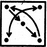and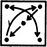alternately.
Series Quiz IV, Non Verbal Reasoning - Question 3

### Directions to Solve Each of the following questions consists of five figures marked A, B, C, D and E called the Problem Figures followed by five other figures marked 1, 2, 3, 4 and 5 called the Answer Figures. Select a figure from amongst the Answer Figures which will continue the same series as established by the five Problem Figures. Question -   Select a figure from amongst the Answer Figures which will continue the same series as established by the five Problem Figures.              Problem Figures:                                                     Answer Figures: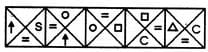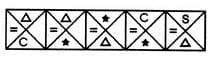(A)     (B)     (C)     ( D)      (E)                               (1)      (2)       (3)       (4)      (5)

Detailed Solution for Series Quiz IV, Non Verbal Reasoning - Question 3

The '=' sign moves to the adjacent position in a clockwise direction in each step. The symbol at the anti clockwise-end position in fig. (A), moves to the adjacent position in an anti clockwise direction in each step and gets replaced by a new symbol in first, third, fifth, ... steps. The symbol at the clockwise-end position in fig. (A), moves to the adjacent position in an anti clockwise direction in each step and gets replaced by a new symbol in second, fourth, sixth, ... steps.

Series Quiz IV, Non Verbal Reasoning - Question 4

Each of the following questions consists of five figures marked A, B, C, D and E called the Problem Figures followed by five other figures marked 1, 2, 3, 4 and 5 called the Answer Figures. Select a figure from amongst the Answer Figures which will continue the same series as established by the five Problem Figures.

Question -

Select a figure from amongst the Answer Figures which will continue the same series as established by the five Problem Figures.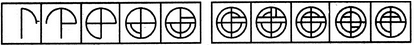(A)     (B)     (C)     (D)     (E)       (1)     (2)     (3)     (4)     (5)
Detailed Solution for Series Quiz IV, Non Verbal Reasoning - Question 4
Two elements are added in each step, be it two lines, two arcs or one line and one arc.
Series Quiz IV, Non Verbal Reasoning - Question 5

Each of the following questions consists of five figures marked A, B, C, D and E called the Problem Figures followed by five other figures marked 1, 2, 3, 4 and 5 called the Answer Figures. Select a figure from amongst the Answer Figures which will continue the same series as established by the five Problem Figures.

Question -

Select a figure from amongst the Answer Figures which will continue the same series as established by the five Problem Figures.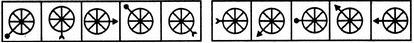(A)     (B)     (C)     (D)     (E)       (1)     (2)     (3)     (4)     (5)
Detailed Solution for Series Quiz IV, Non Verbal Reasoning - Question 5
The arrow moves one, two, three, four, .... spaces ACW sequentially. The arrowhead changes in the sequence: circle -> arc -> triangle -> circle -> ...
Series Quiz IV, Non Verbal Reasoning - Question 6

Each of the following questions consists of five figures marked A, B, C, D and E called the Problem Figures followed by five other figures marked 1, 2, 3, 4 and 5 called the Answer Figures. Select a figure from amongst the Answer Figures which will continue the same series as established by the five Problem Figures.

Question -

Select a figure from amongst the Answer Figures which will continue the same series as established by the five Problem Figures.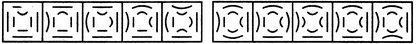(A)     (B)     (C)     (D)     (E)       (1)     (2)     (3)     (4)     (5)
Detailed Solution for Series Quiz IV, Non Verbal Reasoning - Question 6
In each step, all the existing curves get inverted and one of the line segments gets converted to a curve.
Series Quiz IV, Non Verbal Reasoning - Question 7

Each of the following questions consists of five figures marked A, B, C, D and E called the Problem Figures followed by five other figures marked 1, 2, 3, 4 and 5 called the Answer Figures. Select a figure from amongst the Answer Figures which will continue the same series as established by the five Problem Figures.

Question -

Select a figure from amongst the Answer Figures which will continue the same series as established by the five Problem Figures.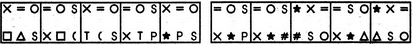(A)     (B)     (C)     (D)     (E)       (1)     (2)     (3)     (4)     (5)
Detailed Solution for Series Quiz IV, Non Verbal Reasoning - Question 7
The symbols move in sequences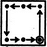and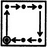alternately. In each step, the symbol that reaches the encircled position gets replaced by a new symbol.
Series Quiz IV, Non Verbal Reasoning - Question 8

Each of the following questions consists of five figures marked A, B, C, D and E called the Problem Figures followed by five other figures marked 1, 2, 3, 4 and 5 called the Answer Figures. Select a figure from amongst the Answer Figures which will continue the same series as established by the five Problem Figures.

Question -

Select a figure from amongst the Answer Figures which will continue the same series as established by the five Problem Figures.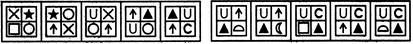(A)     (B)     (C)     (D)     (E)       (1)     (2)     (3)     (4)     (5)
Detailed Solution for Series Quiz IV, Non Verbal Reasoning - Question 8
In the first step, the elements interchange positions in the sequence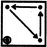. In each subsequent step, the elements move in the sequence obtained by rotating the previous sequence through 90oCW. Also, in each step, the element that reaches the encircled position gets replaced by a new element.
Series Quiz IV, Non Verbal Reasoning - Question 9

Each of the following questions consists of five figures marked A, B, C, D and E called the Problem Figures followed by five other figures marked 1, 2, 3, 4 and 5 called the Answer Figures. Select a figure from amongst the Answer Figures which will continue the same series as established by the five Problem Figures.

Question -

Select a figure from amongst the Answer Figures which will continue the same series as established by the five Problem Figures.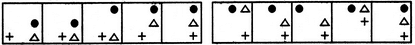(A)     (B)     (C)     (D)     (E)       (1)     (2)     (3)     (4)     (5)
Detailed Solution for Series Quiz IV, Non Verbal Reasoning - Question 9
In the first step, the V sign moves half-a-side of the square boundary in an ACW direction; in the second step, the black circle moves half-a-side of the square boundary in an ACW direction and in the third step, the triangle moves half-a-side of the square boundary in an ACW direction. The three steps are repeated to continue the series.
Series Quiz IV, Non Verbal Reasoning - Question 10

Each of the following questions consists of five figures marked A, B, C, D and E called the Problem Figures followed by five other figures marked 1, 2, 3, 4 and 5 called the Answer Figures. Select a figure from amongst the Answer Figures which will continue the same series as established by the five Problem Figures.

Question -

Select a figure from amongst the Answer Figures which will continue the same series as established by the five Problem Figures.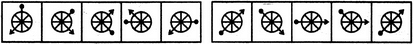(A)     (B)     (C)     (D)     (E)       (1)     (2)     (3)     (4)     (5)
Detailed Solution for Series Quiz IV, Non Verbal Reasoning - Question 10
The pin moves 1, 2, 3, 4, 5, .... spaces CW sequentially. The arrow moves two steps ACW each time.
Series Quiz IV, Non Verbal Reasoning - Question 11

Each of the following questions consists of five figures marked A, B, C, D and E called the Problem Figures followed by five other figures marked 1, 2, 3, 4 and 5 called the Answer Figures. Select a figure from amongst the Answer Figures which will continue the same series as established by the five Problem Figures.

Question -

Select a figure from amongst the Answer Figures which will continue the same series as established by the five Problem Figures.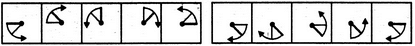(A)     (B)     (C)     (D)     (E)       (1)     (2)     (3)     (4)     (5)
Detailed Solution for Series Quiz IV, Non Verbal Reasoning - Question 11
Similar figure appears in every second step and each time a figure reappears, it rotates 90oCW.
Series Quiz IV, Non Verbal Reasoning - Question 12

Each of the following questions consists of five figures marked A, B, C, D and E called the Problem Figures followed by five other figures marked 1, 2, 3, 4 and 5 called the Answer Figures. Select a figure from amongst the Answer Figures which will continue the same series as established by the five Problem Figures.

Question -

Select a figure from amongst the Answer Figures which will continue the same series as established by the five Problem Figures.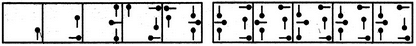(A)     (B)     (C)     (D)     (E)       (1)     (2)     (3)     (4)     (5)
Detailed Solution for Series Quiz IV, Non Verbal Reasoning - Question 12
We shall assign numbers to the pins as :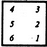. Then, in each step, the pins at positions 1,3 and 5 rotate 90oCW while the pins at positions 2,4 and 6 rotate 90oACW. Also, in each step, a new pin is added to the figure and its orientations are the same as that of the pin in fig. (A).
Series Quiz IV, Non Verbal Reasoning - Question 13

Each of the following questions consists of five figures marked A, B, C, D and E called the Problem Figures followed by five other figures marked 1, 2, 3, 4 and 5 called the Answer Figures. Select a figure from amongst the Answer Figures which will continue the same series as established by the five Problem Figures.

Question -

Select a figure from amongst the Answer Figures which will continue the same series as established by the five Problem Figures.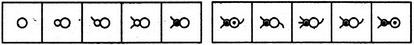(A)     (B)     (C)     (D)     (E)       (1)     (2)     (3)     (4)     (5)
Detailed Solution for Series Quiz IV, Non Verbal Reasoning - Question 13
A new feature is added at each step (in a set order).
Series Quiz IV, Non Verbal Reasoning - Question 14

Each of the following questions consists of five figures marked A, B, C, D and E called the Problem Figures followed by five other figures marked 1, 2, 3, 4 and 5 called the Answer Figures. Select a figure from amongst the Answer Figures which will continue the same series as established by the five Problem Figures.

Question -

Select a figure from amongst the Answer Figures which will continue the same series as established by the five Problem Figures.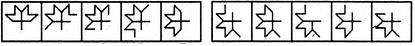(A)     (B)     (C)     (D)     (E)       (1)     (2)     (3)     (4)     (5)
Detailed Solution for Series Quiz IV, Non Verbal Reasoning - Question 14
In each step, one line disappears from the upper part of the figure and one line is added to the lower part of the figure.
Series Quiz IV, Non Verbal Reasoning - Question 15

Each of the following questions consists of five figures marked A, B, C, D and E called the Problem Figures followed by five other figures marked 1, 2, 3, 4 and 5 called the Answer Figures. Select a figure from amongst the Answer Figures which will continue the same series as established by the five Problem Figures.

Question -

Select a figure from amongst the Answer Figures which will continue the same series as established by the five Problem Figures.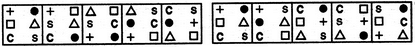(A)     (B)     (C)     (D)     (E)       (1)     (2)     (3)     (4)     (5)
Detailed Solution for Series Quiz IV, Non Verbal Reasoning - Question 15
The symbols move in the sequences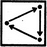and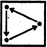alternately.
Series Quiz IV, Non Verbal Reasoning - Question 16

Each of the following questions consists of five figures marked A, B, C, D and E called the Problem Figures followed by five other figures marked 1, 2, 3, 4 and 5 called the Answer Figures. Select a figure from amongst the Answer Figures which will continue the same series as established by the five Problem Figures.

Question -

Select a figure from amongst the Answer Figures which will continue the same series as established by the five Problem Figures.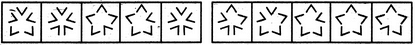(A)     (B)     (C)     (D)     (E)       (1)     (2)     (3)     (4)     (5)
Detailed Solution for Series Quiz IV, Non Verbal Reasoning - Question 16
Two and four 'V' shaped elements get inverted alternately.
Series Quiz IV, Non Verbal Reasoning - Question 17

Each of the following questions consists of five figures marked A, B, C, D and E called the Problem Figures followed by five other figures marked 1, 2, 3, 4 and 5 called the Answer Figures. Select a figure from amongst the Answer Figures which will continue the same series as established by the five Problem Figures.

Question -

Select a figure from amongst the Answer Figures which will continue the same series as established by the five Problem Figures.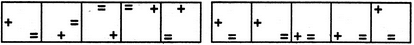(A)     (B)     (C)     (D)     (E)       (1)     (2)     (3)     (4)     (5)
Detailed Solution for Series Quiz IV, Non Verbal Reasoning - Question 17
The V sign moves two spaces (each space is equal to half-a-side of the square boundary) and one space ACW alternately. The '=' sign moves one space and two spaces ACW alternately.
Series Quiz IV, Non Verbal Reasoning - Question 18

Each of the following questions consists of five figures marked A, B, C, D and E called the Problem Figures followed by five other figures marked 1, 2, 3, 4 and 5 called the Answer Figures. Select a figure from amongst the Answer Figures which will continue the same series as established by the five Problem Figures.

Question -

Select a figure from amongst the Answer Figures which will continue the same series as established by the five Problem Figures.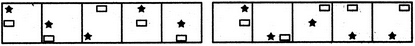(A)     (B)     (C)     (D)     (E)       (1)     (2)     (3)     (4)     (5)
Detailed Solution for Series Quiz IV, Non Verbal Reasoning - Question 18
In each step, both the elements move one space (each space is equal to half-a-side of the square boundary) downwards. Once any of the two elements reaches the lowermost position, then in the next step, it reaches the uppermost position in the next column to the right.
Series Quiz IV, Non Verbal Reasoning - Question 19

Each of the following questions consists of five figures marked A, B, C, D and E called the Problem Figures followed by five other figures marked 1, 2, 3, 4 and 5 called the Answer Figures. Select a figure from amongst the Answer Figures which will continue the same series as established by the five Problem Figures.

Question -

Select a figure from amongst the Answer Figures which will continue the same series as established by the five Problem Figures.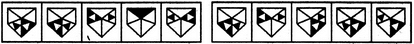(A)     (B)     (C)     (D)     (E)       (1)     (2)     (3)     (4)     (5)
Detailed Solution for Series Quiz IV, Non Verbal Reasoning - Question 19
One of the designs (with white triangle at the centre) moves CW while the other design (with black triangle at the centre) moves ACW.
Series Quiz IV, Non Verbal Reasoning - Question 20

Each of the following questions consists of five figures marked A, B, C, D and E called the Problem Figures followed by five other figures marked 1, 2, 3, 4 and 5 called the Answer Figures. Select a figure from amongst the Answer Figures which will continue the same series as established by the five Problem Figures.

Question -

Select a figure from amongst the Answer Figures which will continue the same series as established by the five Problem Figures.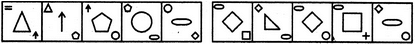(A)     (B)     (C)     (D)     (E)       (1)     (2)     (3)     (4)     (5)
Detailed Solution for Series Quiz IV, Non Verbal Reasoning - Question 20
In each step, the upper element is lost; the middle element reduces in size and becomes the upper element; the lower element enlarges and becomes the middle element and a new element appears at the lower position.

## General Intelligence and Reasoning for SSC CGL

110 videos|66 docs|82 tests
Information about Series Quiz IV, Non Verbal Reasoning Page
In this test you can find the Exam questions for Series Quiz IV, Non Verbal Reasoning solved & explained in the simplest way possible. Besides giving Questions and answers for Series Quiz IV, Non Verbal Reasoning, EduRev gives you an ample number of Online tests for practice

## General Intelligence and Reasoning for SSC CGL

110 videos|66 docs|82 tests(Scan QR code)